# 音视频开发进阶｜图像位深、宽高、跨距# 一、图像位深

• 通道位深：8bit，表示存储色彩空间的一个分量（通道）需要 8bit 空间；

• 像素位深：24bit，表示存储一个 RGB 像素需要 24bit 空间。

• 32bit 图像位深：在 24bit RGB 图像的基础上，增加了一个 8bit 的透明通道 A。比如我们上篇推文提到的 RGBA、BGRA 等等，可以称为 RGBA32、BGRA32；

• 16bit 图像位深：R、G、B 通道分量，分别使用 5bit、6bit、5bit 通道位深 ，可以称为 RGB565；

• 8bit 图像位深：R、G、B 通道分量，分别使用 2bit、3bit、3bit 通道位深，可以称为 RGB233。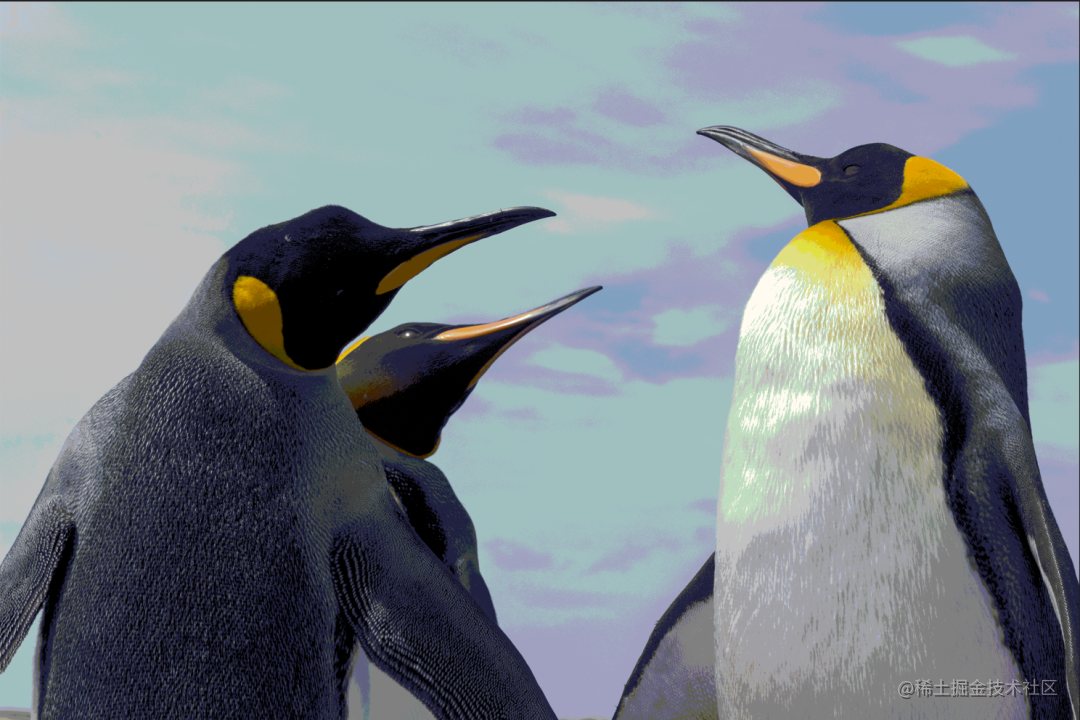bit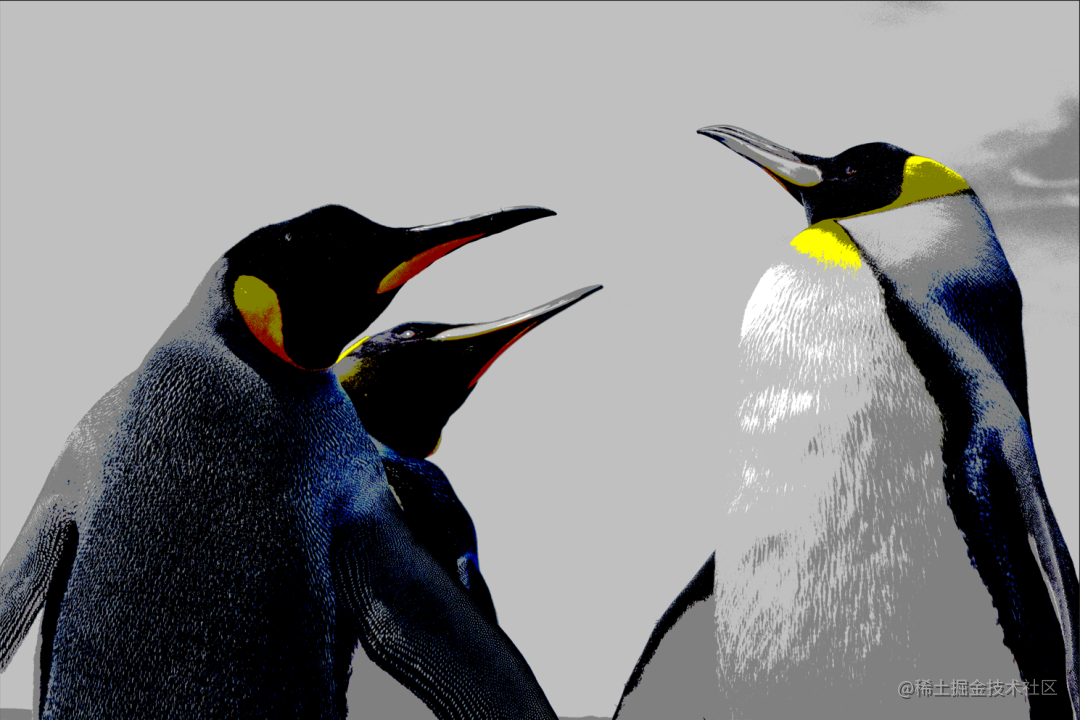# 二、图像宽高（Width、Height）与跨距（Stride）

## 1、图像宽、高

• 宽（Width）：水平方向每行的像素个数，等于图像分辨率的宽

• 高（Height）：垂直方向每列的像素个数，等于图像分辨率的高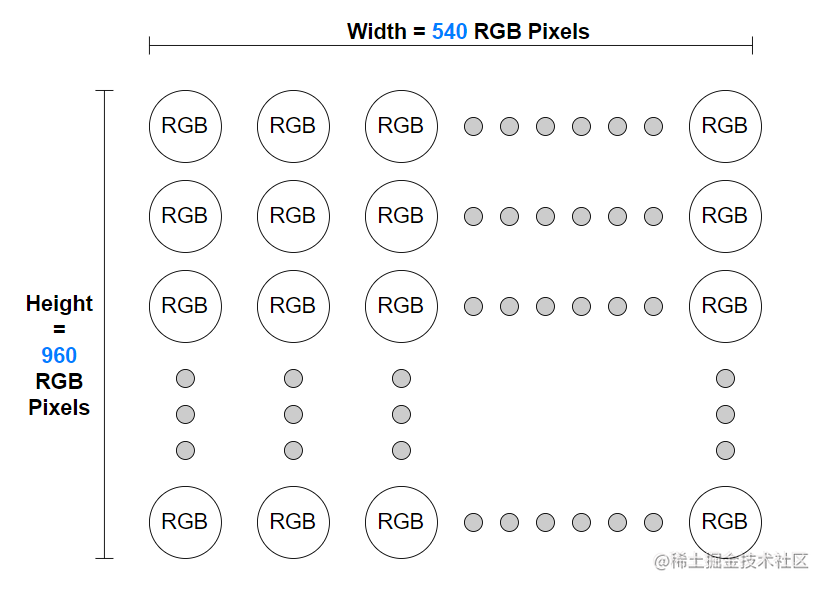• 每行像素的个数 = 图像分辨率宽 = 538

• 每行像素的字节长度 = 像素位深 x 每行像素的个数 = 24 bit x 538 = 1614 byte（ 注：1 byte = 8 bit）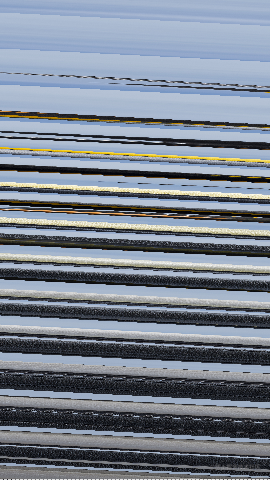## 2、图像跨距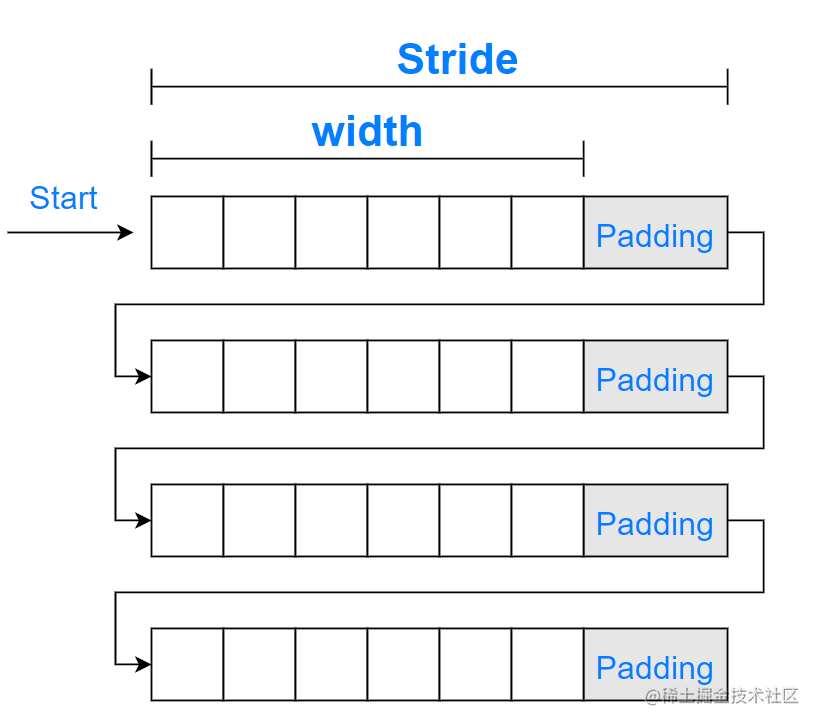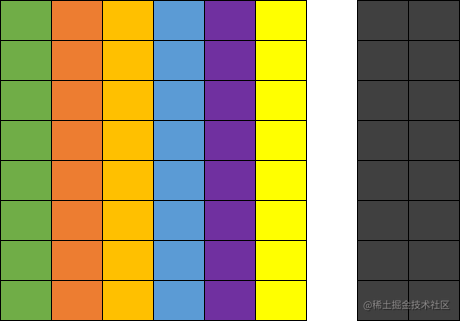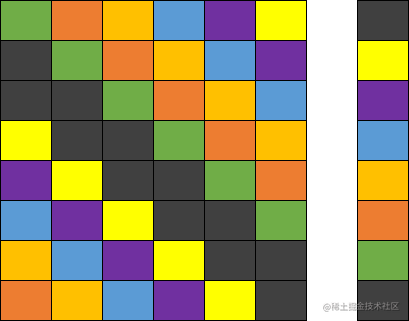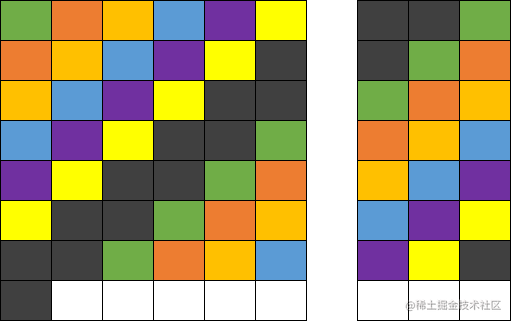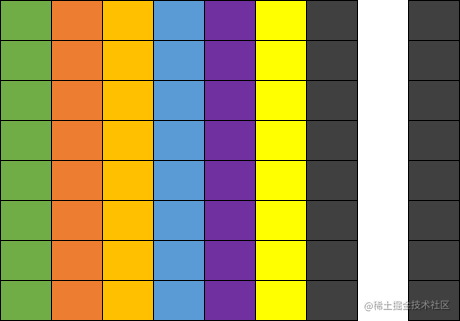## 3、分平面 YUV 的 Width、Stride**

“在每个平面上、每次要读取多少数据”，意味着需要知道每个平面的 Width 和 Stride。而考虑到 U、V 分量相对于 Y 分量可能有降采样，各个分量平面的 Width、Stride 可能不同， 必需要按存储规则分别求取。

• Width_Y_Plane = 每行 Y 分量个数 = 图像每行像素个数 = Width = 720

• Stride_Y_Plane = Width_Y_Plane x 通道位深 = Width_Y_Plane x 8 bit = 720 byte

### 3.1  I422

I422 的采样和存储逻辑简述为：

• 采样：Y 分量全采集，宽度方向每两个 Y 分量共用一组 UV 分量，高度方向每行独立采集 UV 分量

• 存储：Y、U、V 分别存储于三个平面，对于一个宽度为 4 个像素、高度为 2 个像素的采样区域，三个平面分别为 4x2、2x2、2x2 的数组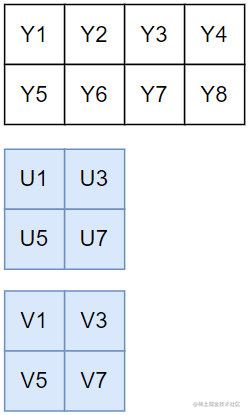• Width_U_Plane = 每行 U 分量个数 = 每行像素个数/2 = Width/2 = 360

• Stride_U_Plane =  Width_U_Plane x 通道位深 = 360 byte

• Stride_V_Plane =  Width_V_Plane x 通道位深 = 360 byte。

### 3.2  I420

I420 的采样和存储逻辑简述为：

• 采样：Y分量全采集，宽度方向和高度方向每四个 Y 分量共用一组 UV 分量，也即第二行复用第一行的 UV 采样；

• 存储：Y、U、V 分别存储于三个平面，对于一个宽度为 4 个像素、高度为 2 个像素的采样区域，三个平面分别为 4x2、2x1、2x1 的数组。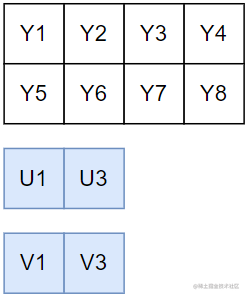• Width_U_Plane = 每行 U 分量个数 = 每行像素个数/2 = Width/2 = 360

• Stride_U_Plane =  Width_U_Plane x 通道位深 = 360byte

• Stride_V_Plane =  Width_V_Plane x 通道位深 = 360byte

### 3.3  NV21

NV21 的采样和存储逻辑简述为：

• 采样：Y分量全采集，宽度方向和高度方向每四个 Y 分量共用一组UV分量，也即第二行复用第一行的 UV 采样

• 存储：Y、UV 分别存储于两个平面，对于一个宽度为 4 个像素、高度为 2 个像素的采样区域，两个平面分别为 4x2、4x1 的数组，UV 共同存储于第二个平面，并按 V、U 的顺序交错存放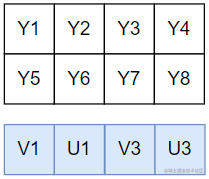• Width_UV_Plane = 每行 U分量个数 + V 分量个数 = 每行像素个数/2 + 每行像素个数/2 =  Width = 720

• Stride_UV_Plane =  Width_UV_Plane x 通道位深 = 720 byte

• Stride_I422 + Stride_I422 + Stride_I422 = Width x 2

• Stride_I420 + Stride_I420 + Stride_I420 = Width x 2

• Stride_NV21 + Stride_NV21 = Width x 2

• Data_I422 = Data_Y + Data_U + Data_V

= Height x Width + Height x Width/2 + Height x Width/2

= Height x Width x 2

• Data_I420 = Data_Y + Data_U + Data_V

= Height x Width + Height/2 x Width/2 + Height/2 x Width/2

= Height x Width x 1.5

• Data_NV21 = Data_Y + Data_UV

= Height x Width + Height/2 x Width

= Height x Width x 1.5

# 三、总结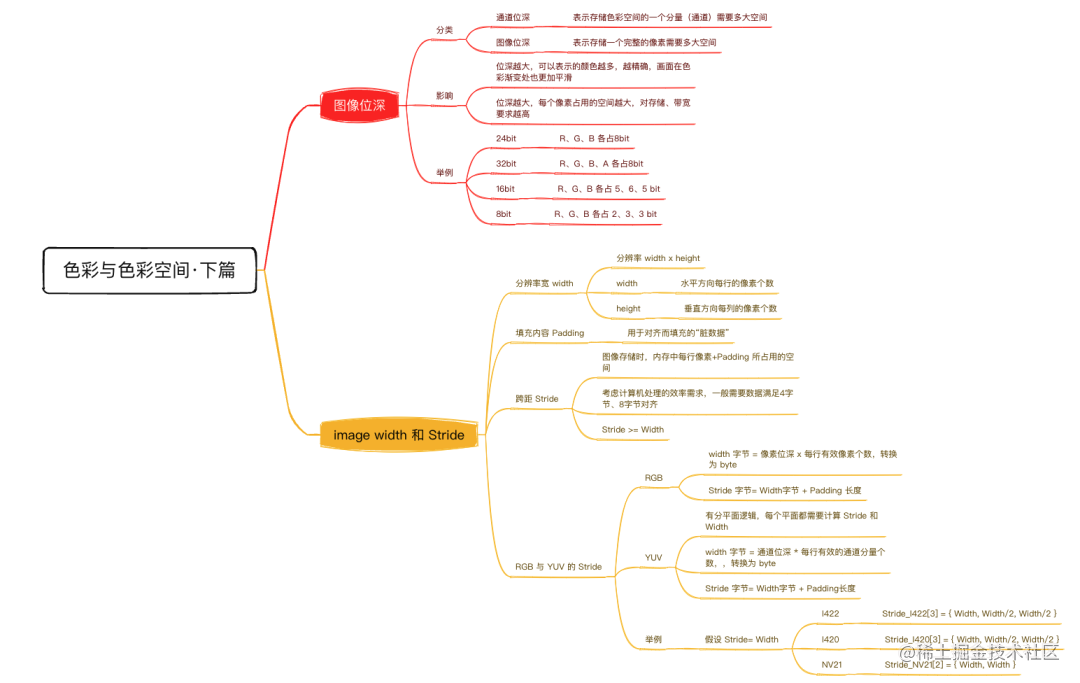（🤫下期揭秘）